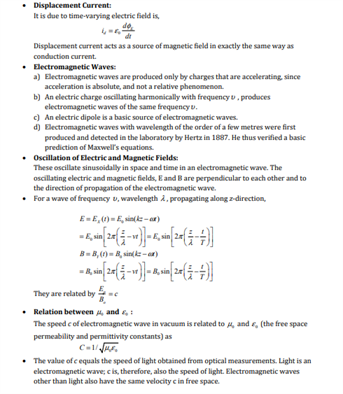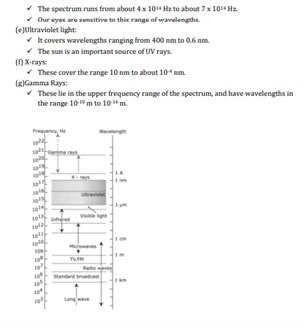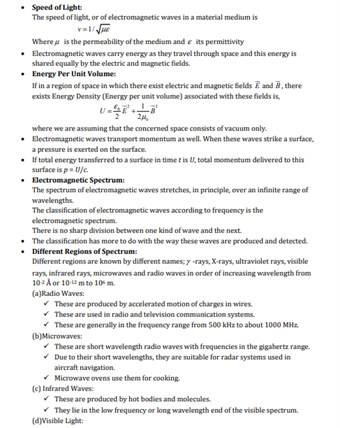# Physics Revision Notes for Class 12 for Chapter 8 Electromagnetic Waves

Maxwell formulated a set of equations involving magnetic and electric field, their sources, charge and current densities. These equations are known as Maxwell’s equations. The most important prediction to emerge from these equations is the existence of electromagnetic waves which are a time-varying electric field and magnetic fields coupled together. In the CBSE class 12 Physics Chapter 8 Electromagnetic waves, the following topics are discussed:

• The need for displacement current and its consequences
• A descriptive account of electromagnetic waves
• The broad spectrum of electromagnetic waves stretching from γ rays ( having a wavelength of 10-12 m) to radios waves ( wavelength of 106 m)

Avail the CBSE class 12 physics revision notes for Chapter 8 Electromagnetic Waves provided below.